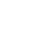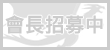LV. 34
GP 357# 《林詩的心願》(９)　火龍與天狗

「又有奇怪的事情發生了……」拉杜疑惑著。

「這樣才好啊！這就代表一定跟火龍有關！」娜芬蒂兒興奮地說。

「娜芬蒂兒喵，還不能確定這一定跟火龍有關吧喵……」林詩覺得，還是先別抱太大希望比較好，免得失望時會更難過。

「好啦！好啦！我只是在幫妳打打氣嘛！」娜芬蒂兒安慰林詩說。

「現在問題是我們應該如何讓那團火熄滅？」拉杜問。

「我聽萊絲說過中國有一個叫做『西遊記』的故事，故事中有一個叫做『火焰山』的地方，那地方就像現在的火龍山一樣。據說後來火焰山山頂的火是故事主角跟一個叫『鐵扇公主』的人借『芭蕉扇』所熄滅的。」娜芬蒂兒回想著萊絲說過的故事。

「拜託！娜芬蒂兒，我們要上哪找那個叫『鐵扇公主』的人借『芭蕉扇』啊？」拉杜有時真的會被娜芬蒂兒的天真所打敗。

「自由操縱風的『天狗扇』？！聽起來好像還真有點相關呢！」拉杜思索著。

「不如我們就照著林詩所說的，去河谷看看能不能遇到這個叫做『天狗』的仙人。」娜芬蒂兒建議著。

「好吧！反正我們也不能繼續前進了，不如先到河谷去看看吧！」拉杜附和著。

=^.^=~=^.^=~=^.^=~=^.^=~=^.^=~=^.^=~=^.^=~=^.^=~=^.^=~=^.^=~=^.^=~=^.^=

「什麼也沒有耶喵……」林詩垂下了雙肩，顯得挺失望的。

「不然我們叫看看好了…喂！天狗！你給我出來！」拉杜用了一個最蠢的方法。他本來只是想逗林詩開心的，沒想到……

「誰在叫我？」天狗就這樣出現在三人面前，害三人差點暈倒。

「呃…是我！」拉杜對天狗說。雖然天狗出場的方式有點「出乎意料」，不過天狗出現就代表他們有可能可以得到「天狗扇」，進一步得到「火龍之鑰」，所以三人也就不再計較天狗如何登場了。

「哦？你找我有什麼事嗎？」天狗看向拉杜。

「是這樣的，我們想得到『天狗扇』！」拉杜直接表明來意。

「哦？那你們可要先打贏我才行！」天狗跟三人提出條件。

「沒問題喵！」林詩信心高漲。

「呵呵呵…這可真有趣，已經好久沒有人來向我挑戰了。好吧！就讓我瞧瞧你們的實力吧！」天狗對三人說。

「呵呵呵…真的很不錯！你們確實有這個能力可以得到這把『天狗扇』，拿去吧！」天狗將一把有紅色的柄，上面插有白色羽毛的扇子交給他們。

「謝謝天狗！」三人異口同聲地說。

「呵呵呵…不客氣！希望你們有空還能來找我比武，陪我磨練磨練筋骨。」說完，天狗就憑空消失了。

「還真是個來無影去無縱的仙人。」拉杜笑道。

=^.^=~=^.^=~=^.^=~=^.^=~=^.^=~=^.^=~=^.^=~=^.^=~=^.^=~=^.^=~=^.^=~=^.^=

「還真的有用喵！」林詩高興地說。

「是火龍！」拉杜驚呼。

「我就說嘛！那團火果然跟火龍有關！」娜芬蒂兒因為自己猜對了而高興著。

「妳是喵？」林詩問著那個由火龍變成的人。

「我是火神伊芙利坦，山上的火是我為了考驗妳這位人貓族的少女而做的。」火神對著林詩說。

「考驗我喵？」林詩指了指自己，不解地問。

「是的，我知道妳想要獲得『火龍之鑰』，那團火就是為了考驗妳是否有資格得到它。如今妳把那團火給熄滅了，那我也可以將『火龍之鑰』交給妳了。」火神將一個鑲有紅寶石的手鐲交給林詩。

「真…真是太謝謝妳了！火神喵！」林詩開心地不知道該說些什麼，只能一直向火神道謝。

「不客氣！『五龍之鑰』妳還差『土龍之鑰』還沒拿到吧！要小心點！」火神說完，就幻化成一團火消失了，只留下充滿疑惑的三人。

(To be continuated……)

=^.^=~=^.^=~=^.^=~=^.^=~=^.^=~=^.^=~=^.^=~=^.^=~=^.^=~=^.^=~=^.^=~=^.^=

(有時候真不了解自己的腦袋是什麼構成的......=.=")face基於日前微軟官方表示 Internet Explorer 不再支援新的網路標準，可能無法使用新的應用程式來呈現網站內容，在瀏覽器支援度及網站安全性的雙重考量下，為了讓巴友們有更好的使用體驗，巴哈姆特即將於 2019年9月2日 停止支援 Internet Explorer 瀏覽器的頁面呈現和功能。

。Google Chrome（推薦）
。Mozilla Firefox
。Microsoft Edge（Windows10以上的作業系統版本才可使用）

face我們了解您不想看到廣告的心情⋯ 若您願意支持巴哈姆特永續經營，請將 gamer.com.tw 加入廣告阻擋工具的白名單中，謝謝 !【教學】